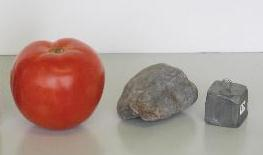How energy is related to temperature

Temperature is an indicator of the presence of a form of energy called thermal energy. When the temperature of an object increases, the amount of thermal energy in it has increased. In order for the temperature of an object or substance to increase, thermal energy must be added to it.

When we add some thermal energy to an object, what happens to it? In most cases it is directly detectable as a small increase in temperature (the exception is during a change in phase). The energy is present in the form of the kinetic energy of the tiny rapid motions of atoms and molecules. In the case of a gas, for which the molecules are far apart, kinetic energy is practically the only form the energy can take. In this case, there is a simple and direct relationship between the energy added, the temperature rise, and the number of atoms.

In a solid or a liquid thermal energy has several forms: The atoms are vibrating back and forth, so they have kinetic energy; but as they move they also are bumping into each other, which involves other kinds of energy. The relationship between energy and temperature is now more complicated, but it is still true that the amount of energy needed to raise the temperature of something is proportional to the size of the temperature increase and to the amount of stuff present.

For example, in the first activity, you placed a large and a small hot rock in cold water. The small rock has a small mass, and so it gained less thermal energy when it was heated to the starting temperature. Then it had less thermal energy to give to the cold water, with the result that the cold water gained less energy -- as shown by the smaller temperature increase caused by the small rock.

Specific Heat and Heat Capacity

The amount of energy needed to raise the temperature of a rock is proportional to the temperature increase and to the size of the rock; it also depends on what the rock is made of. We usually measure "the size of the rock" in terms of its mass (in kilograms). A measurement shows that it takes about 800 Joules to increase the temperature of a particular 1 Kg rock by 1 C o -- we then say that the heat capacity of rock is 800 J/Kg-degree. We can use this result to calculate the amount of energy involved in increasing the temperature of a similar 0.4 kilogram rock by 38 Co: it is just 800 JKg-degree x 0.4 Kg x 38 Co = 12,160 J. (this is equivalent to all the energy stored in a fully charged AA battery, or to the effort of climbing a 6 story building. It's not large -- but it isn't small, either). A smaller rock or a smaller temperature increase would require proportionally less energy, as you found out in the second activity.

For any material, we define the specific heat to be the amount of energy (in Joules) needed to increase the temperature of a 1 Kg sample by 1 Co. Defined this way, the specific heat of materials varies a lot -- it is 4,186 Joules/Kg-degree for water and only 130 Joules/Kg-degree for lead. The reason would be obvious if you had the 1 kilogram samples in front of
tomato, rock, and lead of the same massyou: 1 Kg is a lot of water, but a rather small lump of lead. In fact, lead atoms are very heavy, and so you don't get very many in a kilogram; a kilogram of water contains 35 times as many atoms as a kilogram of lead. The heat capacity of most materials would be rather similar if we were to measure "the size of a rock" in terms of the number of atoms it contains instead of the number of kilograms, and define the specific heat to be the amount of energy needed to raise the temperature of 1 atom (or 1,000,000,000,000,000,000,000,000 atoms, to get a practical kind of unit).

In the third activity involving pennies and tiles, you found that equal masses of copper and ceramic have different abilities to heat air. Assuming that the difference is due to having more atoms, which cup contained more atoms, the one with the pennies or the one with the tiles?

A related concept is heat capacity. This is the amount of energy needed to raise the temperature of an object by 1 Co. An object that contains a lot of atoms has a larger heat capacity than an object containing a small number -- heat capacity is the property of an object, and depends on its size.

In everyday experience, thin stuff like air doesn't have much heat capacity, because there are not many atoms there. This explains how you can run you hand through a candle flame without getting burned, while you definitely will get burned on the oven rack which is much cooler than the flame -- the rack has lots of atoms in it, has a large heat capacity, and can give you lots of thermal energy!

Check the box when you are done: